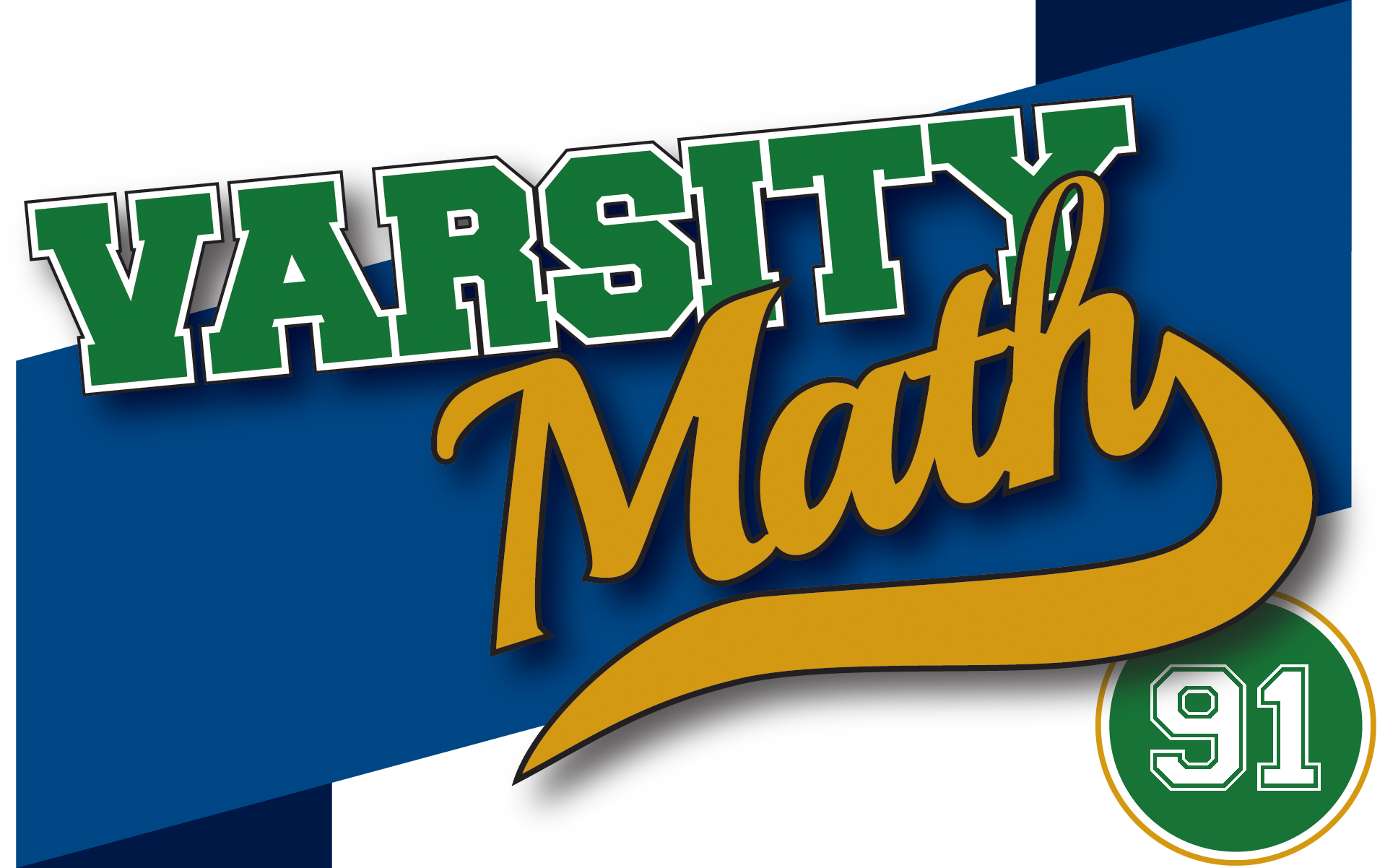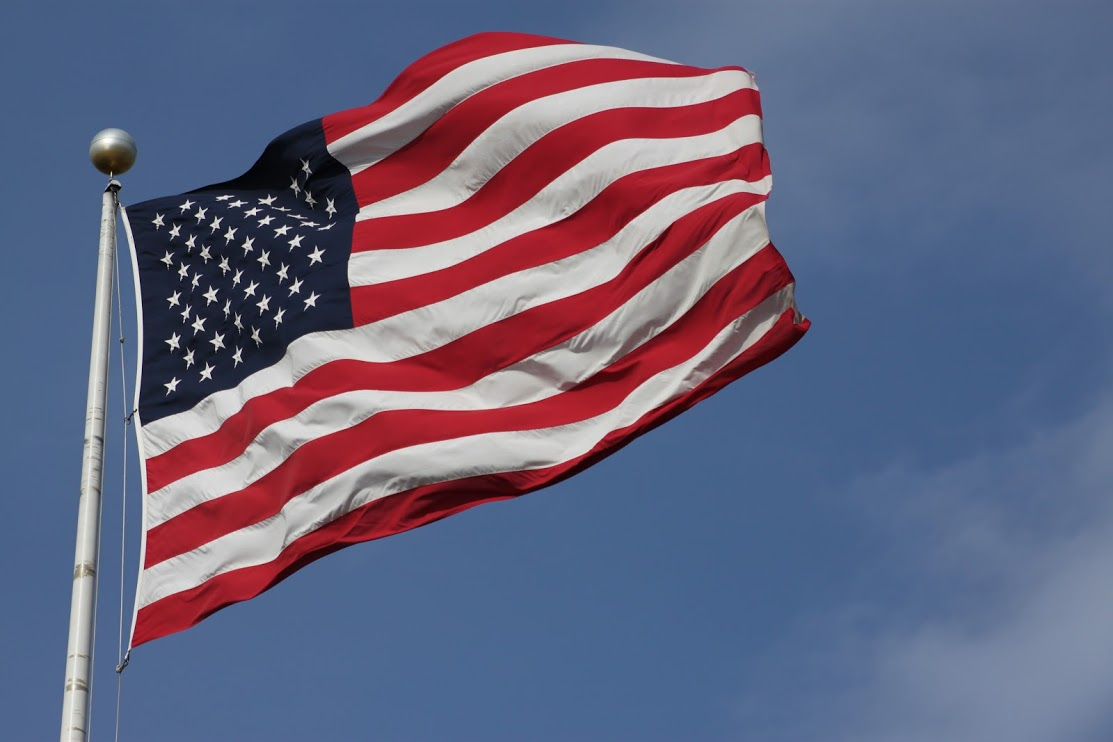## ________________

This week, Coach Taylor has chosen two problems in honor of the upcoming Flag Day.

## ________________

### Hard States

Whenever the number of states in the U.S. has changed, the arrangement of white stars on the blue field of the flag has had to change as well. Say a number s of states is easy if there is an arrangement of s stars into r rows with the following properties: (A) the lengths of any two rows differ by at most one star, (B) if there are two different lengths of rows, no two consecutive rows (from top to bottom) are the same length, and (C) there is a row of stars such that r divided by the number of stars in that row is between 1 and 2, inclusive. For example, 20 states is easy because you can arrange 20 stars into five rows of four, a pattern that satisfies all of the conditions. 50 states is also easy, as our current arrangement of nine rows alternately containing six stars and five stars shows.

What’s the smallest number of states that’s not easy?

### GreatStar

One historic flag has stars arranged in a pentagram, a shape that consists of five points (the vertices) equally spaced around a circle, and five line segments (the edges) connecting each pair of vertices that are not adjacent around the circle. Imagine an arrangement in which you have one star with its center at each vertex of a pentagram, and for each edge of that pentagram, at least one star whose center lies on that edge, not at a vertex. In addition, each edge should have the same number of stars with their centers on it.

What’s the smallest number of stars that can be arranged in this fashion?

## Solutions to week 90Thirds Squared. The diagram shows all of the line segments as specified by the problem, and we are looking for the area of the square with side JK as a fraction of the area of square ABCD. If we let AJ = BK = z, then by similar triangles, AK = 3z and so JK = 2z and its area is 4z². On the other hand, if the side AB is s, then z² + (3z)² = s². So z² = s²/10, so the area of the inner square is 4/10 = 2/5 the area of the outer square.3-4-5 Square. Let s be the side of the square, y be the distance from B to E, and x be the distance from D to F. (See the diagram.) We are given that sy/2 = 3, sx/2 = 4, and (sx)(sy)/2 = 5, and we want to know s². Multiplying the first two equations, we get that xy = 48/s². Multiplying out the third equation, we get that s² – sxsy + xy = 10. Substituting, this is s² – 8 – 6 + 48/s² = 10, and collecting terms and multiplying by s² we get (s²)² – 24s² + 48 = 0. Using the quadratic equation and noting that s² must be positive, we get that the area of the square is 12 + 4√6.

## Recent Weeks

Links to all of the puzzles and solutions are on the Complete Varsity Math page.

Come back next week for answers and more puzzles.

[asciimathsf]